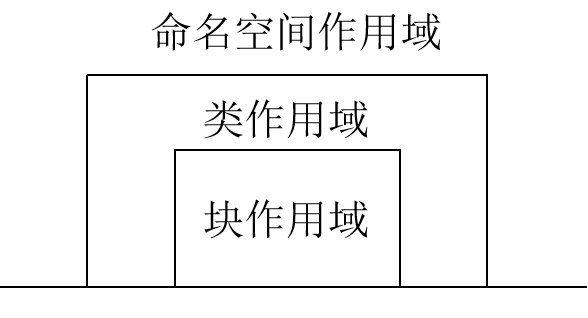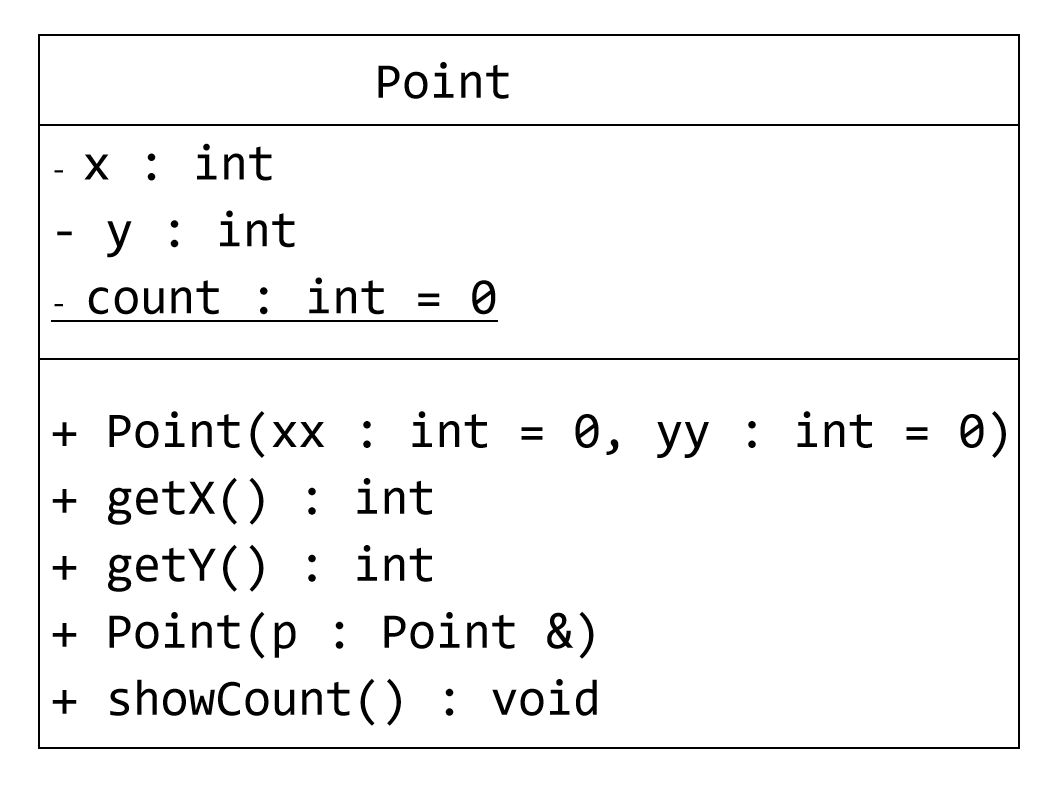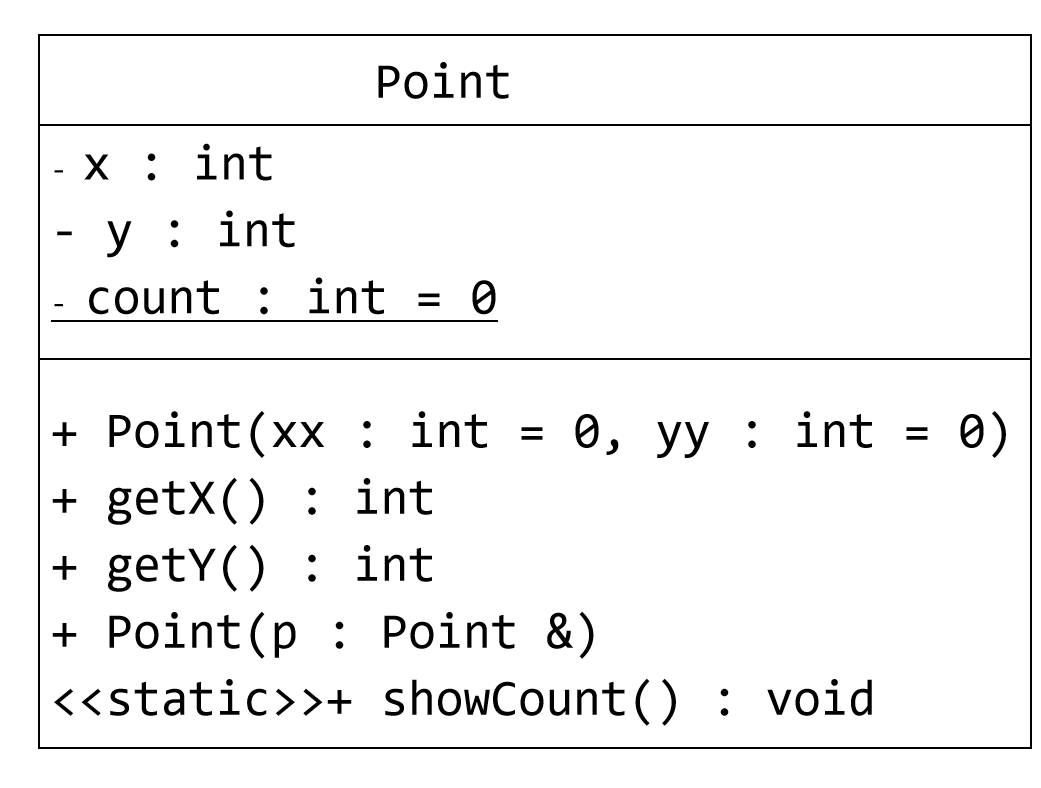C++学习笔记整理-数据的共享与保护

# 第五章数据的共享与保护

## 1.标识符的作用域与可见性

### ①作用域

• 函数原型作用域

• 局部作用域(块作用域)

• 类作用域

• 文件作用域

• 命名空间作用域

//例如，设有下列原型声明：
//radius 的作用域仅在于此area，不能用于程序正文其他地方，因而可有可无。

void fun(int a) {
int b = a;
cin >> b;
if (b > 0) {
int c;

......
}
}

//a的作用域是整个fun函数，b作用域在fun函数内，而c作用域在if语句内

• 类的成员具有类作用域，其范围包括类体和非内联成员函数的函数体。

• 如果在类作用域以外访问类的成员，要通过类名（访问静态成员），或者该类的对象名、对象引用、对象指针（访问非静态成员）。

//example.1
#include
using namespace std;

int i;                //全局变量，文件作用域
int main() {
i = 5;            //为全局变量i赋值
{                //子块1
int i;        //局部变量，局部作用域
i = 7;
cout << "i = " << i << endl;//输出7
}
cout << “i = ” << i << endl;//输出5
return 0;
}


### ②可见性

• 可见性是从对标识符的引用的角度来谈的概念

• 可见性表示从内层作用域向外层作用域“看”时能看见什么。

• 如果标识在某处可见，则就可以在该处引用此标识符。• 标识符应声明在先，引用在后。
• 如果某个标识符在外层中声明，且在内层中没有同一标识符的声明，则该标识符在内层可见。
• 对于两个嵌套的作用域，如果在内层作用域内声明了与外层作用域中同名的标识符，则外层作用域的标识符在内层不可见。

• 在同一作用域内的对象名、函数名、枚举常量名会隐藏同名的类名或枚举类型名。

• 重载的函数可以有相同的函数名。

## 2.对象的生存期

①静态生存期

• 这种生存期与程序的运行期相同。

• 在文件作用域中声明的对象具有这种生存期。

• 在函数内部声明静态生存期对象，要冠以关键字static 。

②动态生存期

• 块作用域中声明的，没有用static修饰的对象是动态生存期的对象（习惯称局部生存期对象）。

• 开始于程序执行到声明点时，结束于命名该标识符的作用域结束处。

//1.变量的生存期与可见性

#include
using namespace std;
int i = 1; // i 为全局变量，具有静态生存期。
void other() {
static int a = 2;
static int b;
// a,b为静态局部变量，具有全局寿命，局部可见。
//只第一次进入函数时被初始化。
int c = 10; // C为局部变量，具有动态生存期，
//每次进入函数时都初始化。
a += 2; i += 32; c += 5;
cout<<"---OTHER---\n";
cout<<" i: "<

## 3.类的静态成员

• 用关键字static声明

• 为该类的所有对象共享，静态数据成员具有静态生存期。

• 必须在类外定义和初始化，用(::)来指明所属的类。//1.具有静态数据成员的Point类

#include
using namespace std;

class Point {    //Point类定义
public:    //外部接口
Point(int x = 0, int y = 0) : x(x), y(y) { //构造函数
//在构造函数中对count累加，所有对象共同维护同一个count
count++;
}
Point(Point &p) {    //复制构造函数
x = p.x;
y = p.y;
count++;
}
~Point() {  count--; }
int getX() { return x; }
int getY() { return y; }

void showCount() {        //输出静态数据成员
cout << "  Object count = " << count << endl;
}
private:    //私有数据成员
int x, y;
static int count;    //静态数据成员声明，用于记录点的个数
};
int Point::count = 0;//静态数据成员定义和初始化，使用类名限定
int main() {    //主函数
Point a(4, 5);    //定义对象a，其构造函数回使count增1
cout << "Point A: " << a.getX() << ", " << a.getY();
a.showCount();    //输出对象个数

Point b(a);    //定义对象b，其构造函数回使count增1
cout << "Point B: " << b.getX() << ", " << b.getY();
b.showCount();    //输出对象个数
return 0;
}

//运行结果：
Point A: 4, 5  Object count=1
Point B: 4, 5  Object count=2

• 类外代码可以使用类名和作用域操作符来调用静态成员函数。
• 静态成员函数只能引用属于该类的静态数据成员或静态成员函数。#include
using namespace std;

class Point {    //Point类定义
public:    //外部接口
Point(int x = 0, int y = 0) : x(x), y(y) { //构造函数
//在构造函数中对count累加，所有对象共同维护同一个count
count++;
}
Point(Point &p) {    //复制构造函数
x = p.x;
y = p.y;
count++;
}
~Point() {  count--; }
int getX() { return x; }
int getY() { return y; }

static void showCount() {        //静态函数成员
cout << "  Object count = " << count << endl;
}

private:    //私有数据成员
int x, y;
static int count;    //静态数据成员声明，用于记录点的个数
};

int Point::count = 0;//静态数据成员定义和初始化，使用类名限定

int main() {    //主函数
Point a(4, 5);    //定义对象a，其构造函数回使count增1
cout << "Point A: " << a.getX() << ", " << a.getY();
Point::showCount();    //输出对象个数

Point b(a);    //定义对象b，其构造函数回使count增1
cout << "Point B: " << b.getX() << ", " << b.getY();
Point::showCount();    //输出对象个数

return 0;
}

## 4.类的友元

转载规则

《C++学习笔记整理-数据的共享与保护》 Tyzhao 采用 知识共享署名 4.0 国际许可协议 进行许可。
目录